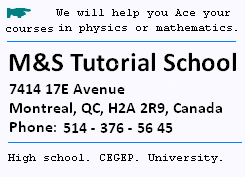Contents# Relativistic Doppler effect

#### 1. Doppler effect for sound

```We have found in the Doppler effect for soud lecture ,
the following results:

```

### 1. Moving source forward an observer at rest

```ƒobs = ƒ . Vw/(Vw+ Vs)

Where ƒobs  is the apparent  frequency for the
Observer, and ƒ is the real frequency of the wave.

```

### 2. Moving source toward an observer at rest

```ƒobs = ƒ . Vw/(Vw- Vs)

ƒobs is the apparent  frequency.

```

### 3. Moving source and observer

```ƒobs = ƒ . (Vw+Vo)/(Vw-Vs)

```

#### 2 Relativistic Doppler effect for light

```According to the dilatation of time, we have
ƒ = 1/T' =  1/γT, hence:

ƒobs = ƒ [(1 + v/c)/(1 - v/c)]1/2
for source and receiver approching, and
ƒobs = ƒ [(1 - v/c)/(1 + v/c)]1/2